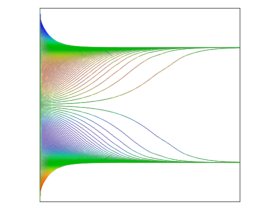# Application Gallery

## Inertial Focusing Between Two Parallel Walls

Application ID: 42951

For more than 50 years, it has been known that neutrally buoyant particles in a flow channel tend to converge to specific locations in the channel cross section. For a cylindrical pipe, or two parallel planes carrying a Poiseuille flow, the equilibrium position is about 0.6 times the pipe radius, or a distance from parallel walls of about 0.2 times the channel width, respectively. This is called the Segre-Silberberg effect, while a ring of particles with a radius of 0.6 times the pipe radius is sometimes called the Segre-Silberberg annulus.

In this benchmark model, we reproduce the case of a flow channel bounded by two parallel walls. Wall-dependent lift and drag forces are exerted on the neutrally buoyant particles as they are carried along the channel by a parabolic fluid velocity profile. As particles are carried through the channel, the inertial lift force causes them to reach equilibrium positions at distances from the center of 0.3 D, where D is the distance between the walls. These equilibrium positions are consistent with the Segre-Silberberg effect.This model example illustrates applications of this type that would nominally be built using the following products: